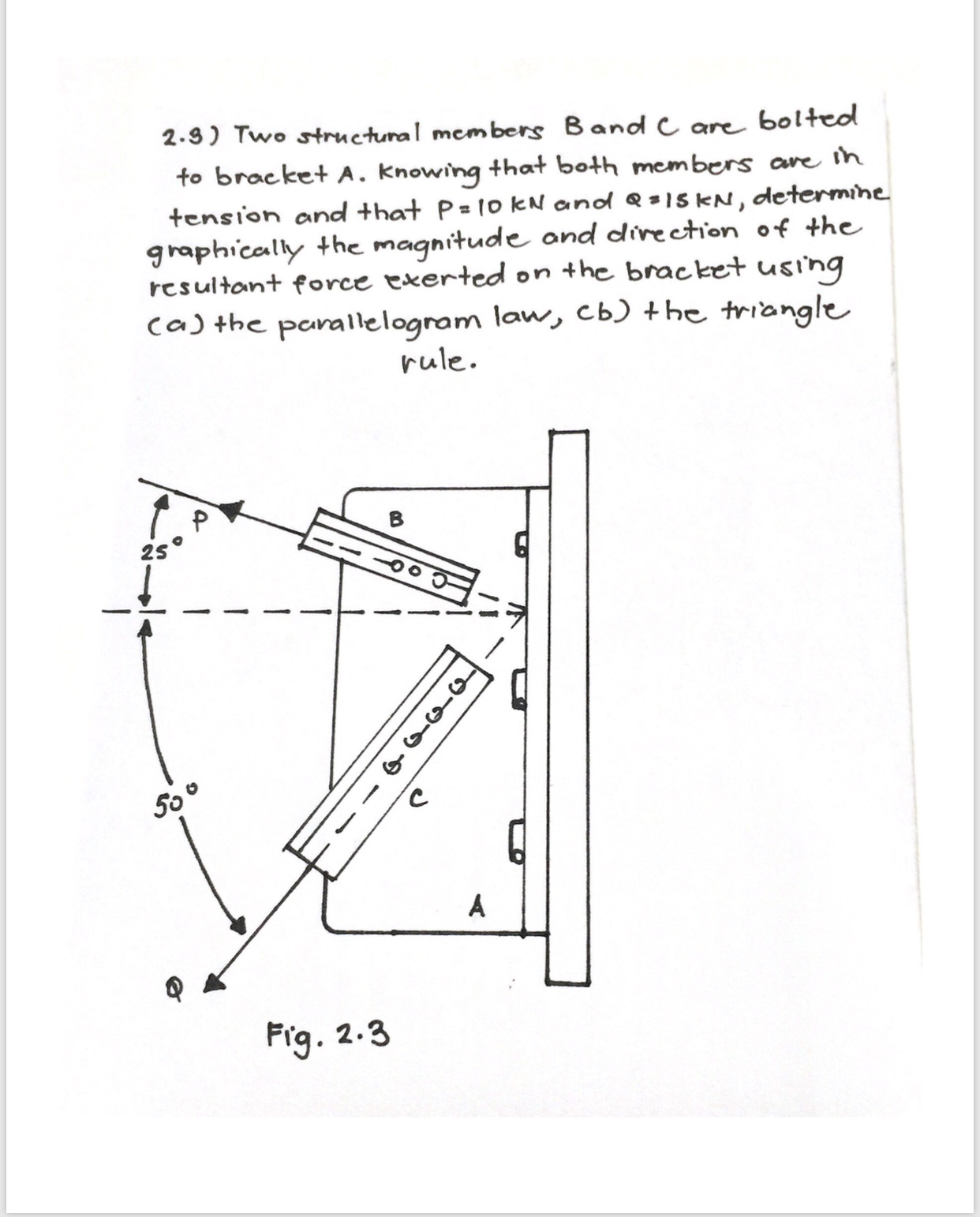# bolteo2.9) Two structunal members Band C areto bracket A. knowing that both members are ihtension and that P= 1o kN andd = 15 KN, determinegraphically the magnitude and direction of theresultant force exerted on the bracket usingcaj the parallelogram law, cb) +he trianglerule2SAFrg2.3o-n-q

Question
3 viewshelp_outlineImage Transcriptionclosebolteo 2.9) Two structunal members Band C are to bracket A. knowing that both members are ih tension and that P= 1o kN andd = 15 KN, determine graphically the magnitude and direction of the resultant force exerted on the bracket using caj the parallelogram law, cb) +he triangle rule 2S A Frg 2.3 o-n-q fullscreen
check_circle

Step 1

To determine: The magnitude and direction of the resultant force.

• Parallelogram law
• Triangle rule.
Step 2

Given:

The magnitude of force P is 10 kN.

The magnitude of force Q is 15 kN.

Step 3

Calculation:

Part(a)

Parallelogram’s law: When two vector P and Q shows the adjacent side of a parallelogram in both the direct...

### Want to see the full answer?

See Solution

#### Want to see this answer and more?

Solutions are written by subject experts who are available 24/7. Questions are typically answered within 1 hour.*

See Solution
*Response times may vary by subject and question.
Tagged in

### Mechanics